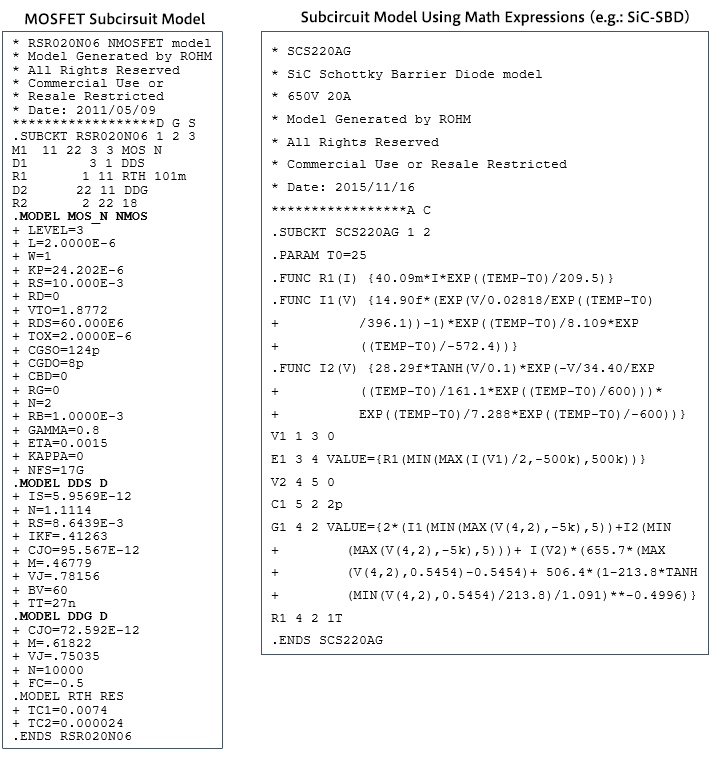Technical Information Site of Power Supply Design

2019.07.24 Simulation

# SPICE Subcircuit Models: Models Using Mathematical Expressions

Foundations of Electronic Circuit Simulation

This article addresses subcircuit models that use mathematical expressions. Here, in addition to the previously explained subcircuit models that combine device modes, it should be remembered that there are also subcircuit models that are based on mathematical expressions.

Subcircuit Models Using Mathematical Expressions

There are also subcircuit models that present device characteristics represented using mathematical expressions or equations. The "MOSFET subcircuit models" explained in the previous article and the article before that were basically configured as a combination of device models; the subcircuit model type considered here combines mathematical expressions. Below are shown examples of a SiC SBD (Schottky barrier diode) subcircuit model of the type that uses mathematical expressions, and of the MOSFET subcircuit model that was previously described. Details are here omitted, but the reader should be able to grasp the differences in the model descriptions.In a subcircuit model that uses mathematical expressions, the expressions can be adjusted in accordance with the device characteristics, and so such models feature high reproducibility. However, such models are complex, and so have the disadvantages of longer simulation times and a tendency for convergence errors to occur.

#### Key Points:

・In addition to subcircuit models that combine device models, there are also subcircuit models based on mathematical expressions.

・The mathematical expressions can be adjusted in keeping with device characteristics, so that high reproducibility is obtained.

・Models are complex, and so simulation times are longer, and convergence errors tend to occur.